# Reduced Basis Methods

## 1. Model Order Reduction

### 1.1. Definition

#### 1.1.1. Problem statement

Goal : Replicate input-output behavior of large-scale system $\Sigma$ over a certain (restricted) range of

• forcing inputs and

• parameter inputs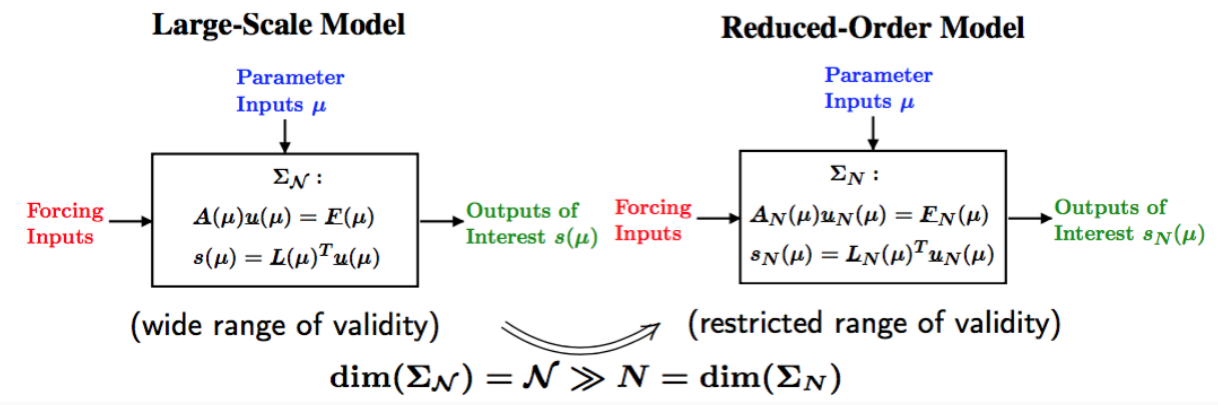Figure 1. Problem statement

Given large-scale system $\Sigma_\mathcal{N}$ of dimension $\mathcal{N}$, find a reduced order model $\Sigma_N$ of dimension $N << \mathcal{N}$ such that: The approximation error is small, i.e., there exists a global error bound such that :

• $\|u(\mu) - u_N (\mu)\| \leq \varepsilon_{\mathrm{des}}$, and $|s(\mu) - s_N (\mu)| \leq \varepsilon^s_{\mathrm{des}} , \forall \mu \in D^{\mu}$.

• Stability (and passivity) is preserved.

• The procedure is computationally stable and efficient.

### 1.2. Motivation

Generalized Inverse Problem :

• Given PDE($\mu$) constraints, find value(s) of parameter $\mu$ which:

• (OPT) minimizes (or maximizes) some functional;

• (EST) agrees with measurements;

• (CON) makes the system behave in a desired manner;

• or some combination of the above

• Full solution computationally very expensive due to a repeated evaluation for many different values of $\mu$

• Goal: Low average cost or real-time online response.

### 1.3. Methodologies

• Reduced Basis Methods

• Proper Orthogonal Decomposition

• Balanced Truncation

• Krylov Subspace Methods

• Proper Generalized Decomposition

• Modal Decomposition

• Physical model reduction

• …​

 Disclaimer on Model Order Reduction Techniques They Do not replace your favorite discretization scheme (e.g. FE, FV, FD), but instead are build upon and supplement these schemes. They are not useful if you are interested in a single high-fidelity solution of your high-dimensional problem, but instead if you are interested in the many-query or real-time context.

### 1.4. Summary

Many problems in computational engineering require many or real-time evaluations of PDE($\mu$)-induced + input-output relationships.

Model order reduction techniques enable certified, real-time calculation + of outputs of PDE($\mu$) + for parameter estimation, optimization, and control.

## 2. Reduced Basis Method

### 2.1. Problem Statement

A model order reduction technique that allows efficient and reliable reducedorder approximations for a large class of parametrized partial differentialequations (PDEs) in real-time or in the limit of many queries.

• Comparison to other model reduction techniques:

• Parametrized problems(material, constants, geometry,…​)

• A posteriori error estimation

• Offline-online decomposition

• Greedy algorithm (to construct reduced basis space)

• Motivation :

• Efficient solution of optimization and optimal control problems governed by parametrized PDEs.

#### 2.1.1. Problem statement

Given a parameter $\underbrace{\mu}_{\text{parameter}} \in \underbrace{D^\mu}_\text{parameter domain}$, evaluate $\underbrace{s(\mu)}_\text{output} = L(\mu)^T \underbrace{u(\mu)}_\text{field variable}$ where $u(\mu)\in\underbrace{X}_\text{FE space}$ satisfies a PDE $A(\mu) u(\mu) = f(\mu)$.

Difficulties :

• Need to solve $\textit{PDE}_{FE}(\mu)$ numerous times at different values of $\mu$,

• Finite element space $X$ has a large dimension $\mathcal{N}$.

#### 2.1.2. Key Observation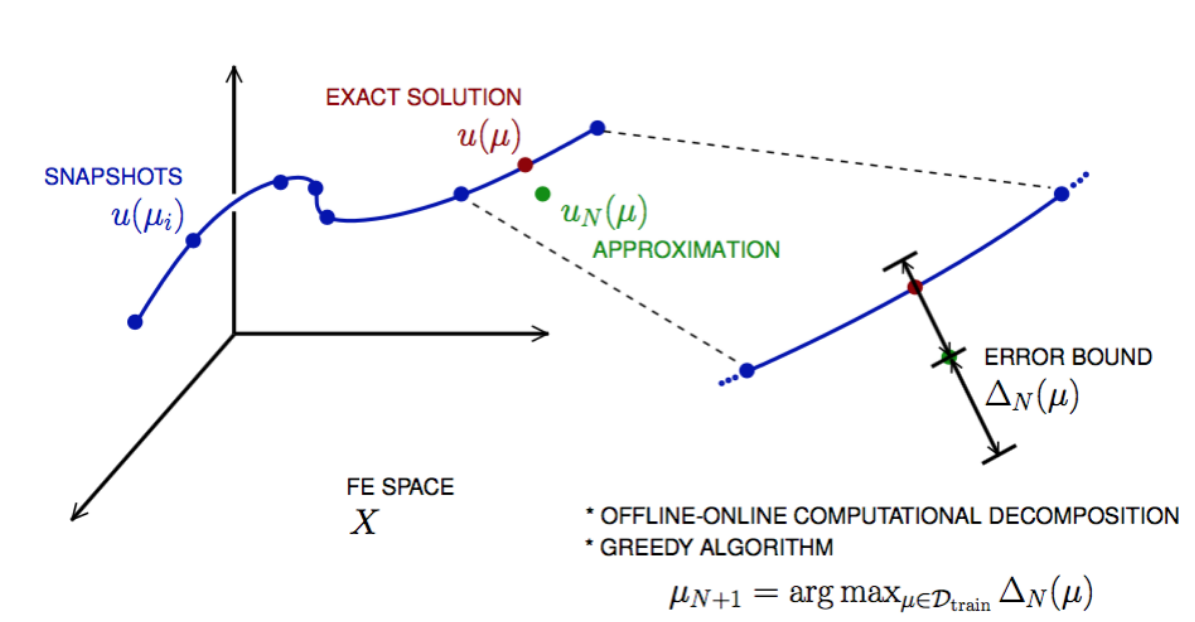Figure 2. Reduced Basis Method

#### 2.1.3. General Problem Statement

Given a system $\Sigma_\mathcal{N}$ of large dimension N,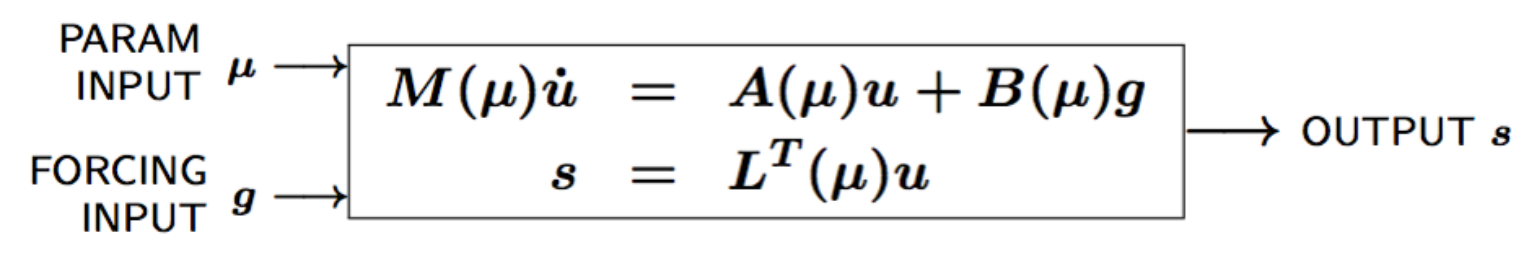Figure 3. Reduced Basis Method

where

• $u(\mu, t) \in \mathbb{R}^{\mathcal{N}}$, the state

• $s(\mu, t)$, the outputs of interest

• $g(t)$, the forcing or control inputs

are functions of

• $\mu \in D$, the parameter inputs

• $t$, time

and the matrices $M$, $A$, $B$, and $L$ also depend on $\mu$.

Construct a reduced order system $\Sigma_N$ of dimension $N \ll \mathcal{N}$,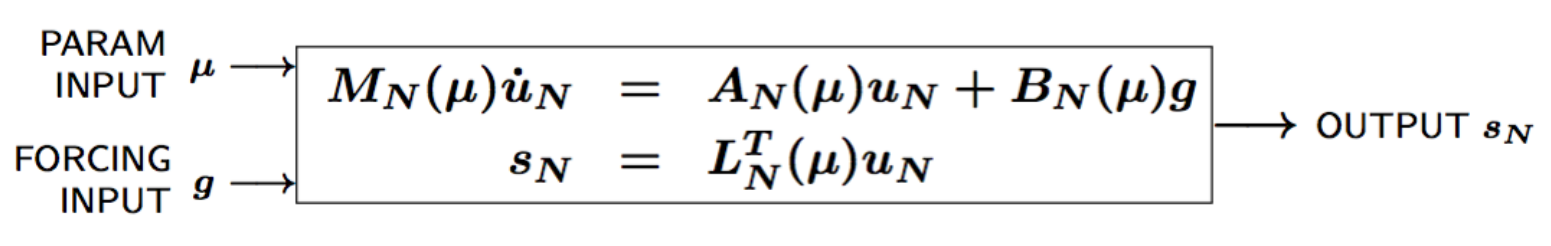Figure 4. Reduced Basis Method

where $u_N(\mu) \in \mathbb{R}^N$ is the reduced state.

We start by considering $\dot{u} = 0$

Full Model

\begin{align} A(\mu) u(\mu)& = & F(\mu)\\ s(\mu)&=&L^T(\mu) u(\mu) \end{align}

Reduced Model

\begin{align} A_N(\mu) u_N(\mu)& = & F_N(\mu)\\ s_N(\mu)&=&L^T_N(\mu) u_N(\mu) \end{align}

### 2.2. Key ingredients

#### 2.2.1. Approximation

• Take « snapshots » at different $\mu$-values: $u(\mu_i), i = 1 \ldots N$, and let $Z_N=[\xi_1,\ldots,\xi_N] \in \mathbb{R}^{\mathcal{N}\times N}$ where the basis/test functions, $\xi_i$ « $=$ » $u(\mu_i)$, are orthonormalized

• For any new $\mu$, approximate $u$ by a linear combination of the $\xi_i$ $u(\mu) \approx \sum_{i=1}^N u_{N,i}(\mu) \xi_i = Z_N u_N(\mu)$ determined by Galerkin projection, i.e.,

\begin{align*} \underbrace{Z_N^T A(\mu) Z_N}_{=:A_N(\mu)}u_N(\mu) &= \underbrace{Z_N^TF(\mu)}_{=:F_N(\mu)}\\ s_N(\mu) &= \underbrace{L^T(\mu)Z_N}_{=:L_N^T(\mu)}u_N(\mu) \end{align*}

#### 2.2.2. A posteriori error estimation

• Assume well-posedness; $A(\mu)$ positive and definite with a minimal eigenvalue $\alpha_a :=\lambda_1 >0$, where $A \xi=\lambda X \xi$ and $X$ corresponds to the $X$-inner product, $(v, v)_X = \|v\|_X^2$

• Let $\underbrace{e_N = u - Z_N\ u_N}_{\text{error}}$ , and $\underbrace{r = F - A\ Z_N\ u_N}_{\text{residual}}, \forall \mu \in D^\mu$, so that $A(\mu) e_N (\mu) = r(\mu)$

• Then (LAX-MILGRAM) for any $\mu \in D^\mu$, $\|u(\mu)- Z_N u_N(\mu) \|_X \leq \frac{\|r(\mu)\|_{X'}}{\alpha_{LB}(\mu)} =: \Delta_N(\mu)$, $|s(\mu)-s_N(\mu)| \leq \|L\|_{X'} \Delta_N(\mu) =: \Delta^s_N(\mu)$ where $\alpha_{LB}(\mu)$ is a lower bound to $\alpha_a(\mu)$, and $\|r\|_{X'}=r^T X^{-1} r$.

#### 2.2.3. Offline-Online decomposition

How do we compute $u_N$, $s_N$, $\Delta_N^s$ for any $\mu$ efficiently online ?

We assue $A(\mu) = \displaystyle\sum_{q=1}^Q \theta^q(\mu)A^q$ where

• $\theta^q(\mu)$ are parameter depandent coefficients,

• $A^q$ are parameter independent matrices

so that $A_N(\mu) = Z_N^T A(\mu)Z_N = \displaystyle \sum_{q=1}^Q \theta^q(\mu)\underbrace{Z_N^T A^q Z_N}_\text{parameter independant}$

Since all required quantities can be decomposed in this manner, we can split the process in two phases :

• OFFLINE : Form and store $\mu$-independant quantities at cost $O(\mathcal{N})$,

• ONLINE : For any $\mu\in D^\mu$, compute approximation and error obunds at cost $O(QN^2+N^3)$ and $O(Q^2N^2)$.

#### 2.2.4. Greedy Algorithm

How do we choose the sample points $\mu_i$ (snapshots) optimally ?

Given $Z_{N=2} « = » [u(\mu_1), u(\mu_2)]$, we choos $\mu_{N+1}$ as follows

$\mu_{N+1} = \mathrm{argmax}_{\mu\in D^\text{train}}\dfrac{\Delta_N(\mu)}{\Vert u_N(\mu)\Vert_X}$

and $Z_{N+1} := [u(\mu_1),\cdots, u(\mu_{N+1})$.

• Key : $\Delta_N(\mu)$ is sharp and inexpensive to compute (online)

• Error bound gives « optimal » samples, so we get a good approximation $u_N(\mu)$.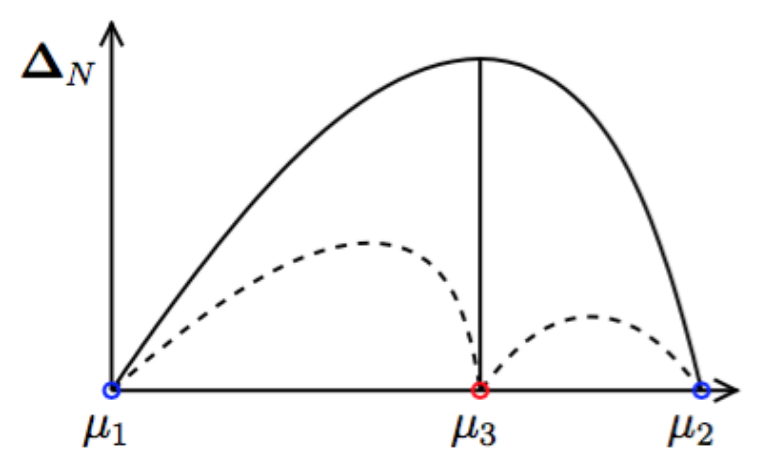Figure 5. Greedy algorithm

### 2.3. Summary

#### 2.3.1. Reduced Basis Opportunities Computational Opportunities

• We restrict our attention to the typically smooth and low-dimensional manifold induced by the parametric dependence.
$\Rightarrow$ Dimension reduction

• We accept greatly increased offline cost in exchange for greatly decreased online cost.
$\Rightarrow$ Real-time and/or many-query context

#### 2.3.2. Reduced Basis Relevance

Real-Time Context (control,$\ldots$): \begin{align} \mu & \rightarrow & s_N(\mu), \Delta^s_N(\mu) & \\ t_0 \text{(« input »)} & & & t_0+\delta t_{\mathrm{comp}} (\text{« response »}) \end{align}

Many-Query Context (design,$\ldots$): \begin{align} \mu_j & \rightarrow & s_N(\mu_j), \Delta^s_N(\mu_j),\quad j=1\ldots J \\ t_0 & & t_0+\delta t_{\mathrm{comp}} J\quad (J \rightarrow \infty) \end{align}

$\Rightarrow$ Low parginal (real-time) and/or low average (many-query) cost.

#### 2.3.3. Reduced Basis Challenges

• A Posteriori error estimation

• Rigorous error bounds for outputs of interest

• Lower bounds to the stability « constants »

• Offline-online computational procedures

• Full decoupling of finite element and reduced basis spaces

• A posteriori error estimation

• Nonaffine and nonlinear problems

• Effective sampling strategies

• High parameter dimensions

#### 2.3.4. Reduced Basis Outline

1. Affine Elliptic Problems

• (non)symmetric, (non)compliant, (non)coercive

• (Convection)-diffusion, linear elasticity, Helmholtz

2. Affine Parabolic Problems

• (Convection)-diffusion equation

3. Nonaffine and Nonlinear Problems

• Nonaffine parameter dependence, nonpolynomial nonlinearities

4. Reduced Basis (RB) Method for Fluid Flow